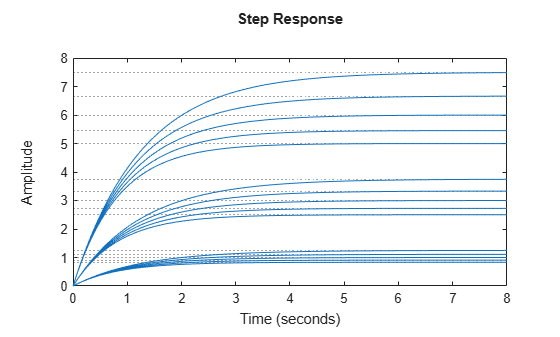sampleBlock

Sample Control Design blocks in generalized model

Description

example

Msamp = sampleBlock(M,name,vals) samples one Control Design block in the generalized model M. The result Msamp is a model array of size [size(M) N] obtained by replacing the block with the specified values, where N is the number of values in vals.

example

Msamp = sampleBlock(M,nameset,valset) concurrently samples multiple blocks specified as a cell array of block names. valset is a cell array of N sample values for each block. The result Msamp is a model array of size [size(M) N].

example

Msamp = sampleBlock(M,nameset1,valset1,nameset2,valset2,...,namesetM,valsetM) independently samples multiple blocks. nameset1,nameset2,...,namesetM can each be a single block name (see name) or a cell array of names (see nameset). The model M is sampled over a grid of size [N1 N2 ... NM], where N1 is the number of values in valset1, N2 is the number of values in valset2, and so on. The resulting Msamp is an array of size [size(M) N1 N2 ... NM].

[Msamp,samples] = sampleBlock(___) also returns a data structure containing the block replacement values for each sampling point. You can use this syntax with any of the preceding input argument combinations.

Examples

collapse all

Create the first-order model $G\left(s\right)=1/\left(\tau s+1\right)$, where $\tau$ is a tunable real parameter.

tau = realp('tau',5);
G = tf(1,[tau 1]);

Evaluate this transfer function for $\tau$ = 3,4,..,7. The result is a 5-by-1 array of first-order models.

Gs = sampleBlock(G,'tau',3:7);
size(Gs)
5x1 array of state-space models.
Each model has 1 outputs, 1 inputs, and 1 states.

Create a model with a pole at s = a and a gain of b*c, where a, b, and c are tunable scalars.

a = realp('a',1);
b = realp('b',3);
c = realp('c',1);
G = tf(b*c,[1 a]);

Pick 5 samples for a and 3 samples for (b,c) pairs. Evaluate G over the corresponding 5-by-3 grid of (a,b,c) combinations.

as = 0.8:0.1:1.2;
bs = 2:4;
cs = [0.5 1 1.5];
Gs = sampleBlock(G,'a',as,{'b','c'},{bs,cs});

Grouping the values for b and c in cell arrays causes sampleBlock to treat them as the (b,c) pairs, (2,0.5), (3,1), and (1,5). Gs is a 5-by-3 array of state-space models, in which a varies along the first dimension and (b,c) varies along the second dimension. Thus, for example, Gs(:,:,3,2) corresponds to a = 1, (b,c) = (3,1).

A step plot shows a set of responses for each of the three (b,c) pairs. Each set contains a response for each of the five a values.

stepplot(Gs)If you do not group the values, sampleBlock replaces all values independently, resulting in a 5-by-3-by-3 model array.

GsInd = sampleBlock(G,'a',as,'b',bs,'c',cs);
size(GsInd)
5x3x3 array of state-space models.
Each model has 1 outputs, 1 inputs, and 1 states.

For example, in GsInd, Gs(:,:,3,2,1) is a model with a = 1, b = 3, and c = 0.5.

Input Arguments

collapse all

Model to sample, specified as a:

• Generalized model (genss or genfrd)

• Generalized matrix (genmat)

• Uncertain model (uss (Robust Control Toolbox) or ufrd (Robust Control Toolbox))

• Uncertain matrix (umat (Robust Control Toolbox))

Control Design block to sample, specified as a character vector. For example, suppose that M is a genss model with tunable blocks t1 and t2. Then, either 't1' or 't2' is a possible value for name.

Sample block values, specified as a numeric array or a model array. Values must be compatible with the block type. For example, if name is a tunable real parameter (realp), then vals is a numerical array of length N, the number of samples. If name is a tunable PID controller (tunablePID), then vals is an array of LTI models compatible with PID structure.

Control Design blocks to sample concurrently, specified as cell array of character vectors. The entries in nameset correspond to the names of at least a subset of the Control Design blocks in M. For example, suppose that M is a genss model with tunable blocks t1 and t2, and uncertain blocks u1 and u2. Then, {'t1','u2'} is one possible value for nameset.

Grouping block names together in a cell array generates samples of the group rather than independent samples. For example, the following code generates a 10-by-1 array of models, where each entry in the array has the corresponding value for the pair (t1,u2).

t1s = 1:10;
u2s = 2:2:20;
valset = {t1s,t2s};
Msamp = sampleBlock(M,{'t1','u2'},valset);

sampleBlock ignores any entry in nameset that does not appear in M.

Sample block values, specified as a cell array. Each entry in the cell array is itself an array of N sample values for each block in nameset. For example, the following code samples a model M at the (t1,u2) pairs (1,2), (2,4), ... (10,20).

t1s = 1:10;
u2s = 2:2:20;
valset = {t1s,t2s};
Msamp = sampleBlock(M,{'t1','u2'},valset);

Values in valset must be compatible with the corresponding block type.

Output Arguments

collapse all

Array of model samples, returned as a generalized model array, ss array, frd array, or numeric array. Msamp is of the same type as M, unless all blocks are sampled. In that case, Msamp is a numeric array, ss array, or frd array. For example, suppose that M is a uss model with uncertain blocks u1 and u2. The following command returns an array of uss models, with uncertain block u2.

Msamp1 = sampleBlock(M,'u1',1:10);

The following command samples both blocks and returns an array of ss models.

Msamp2 = sampleBlock(M,{'u1','u2'},{1:10,2:20});

Block sample values, returned as a structure. The fields of samples are the names of the sampled blocks. The values are arrays containing the corresponding values used to generate the entries in Msamp.